+关注继续查看

什么是指针//
//  main.c
//  Point
//
//  Created by Kenshin Cui on 14-7-05.
//

#include <stdio.h>

int main(int argc, const char * argv[]) {

int a=1;
int *p;
p=&a; //也可以直接给指针变量赋值：int *p=&a;
printf("a=%d,p=%d\n",a,*p); //结果：a=1,p=1
*p=2;
printf("a=%d,*p=%d\n",a,*p); //结果：a=2,p=2

int b=8;
char c= 1;
int *q=&c;
printf("c=%d,q=%d\n", c, *q); //结果：c=1,q=2049，为什么q的值不是1呢？

return 0;
}

a.int *p;中的*只是表示p变量是一个指针变量；而打印*p的时候，*p中的*是操作符，表示p指针指向的变量的存储空间（当前存储就是1），同时我们也看到了*p==a；修改了*p也就是修改了p指向的存储空间的内容，也就修改了a，所以第二次打印a=2;

b.指针所指向的类型必须和定义指针时声明的类型相同；上面指针q定义成了int型而指向了char型，结果输出*q打印出了2049，具体原因见下图（假设在16位编译器下，指针长度为2字节）c.指针变量占用的空间和它所指向的变量类型无关，只跟编译器位数有关（准确的说只跟寻址方式有关）；

数组和指针

//
//  main.c
//  Point
//
//  Created by Kenshin Cui on 14-7-05.
//

#include <stdio.h>

void changeValue(int a[]){
a=2;
}
void changeValue2(int *p){
p=3;
}

int main(int argc, const char * argv[]) {
int a[]={1,2,3};
int *p=&a; //等价于：*p=a;

printf("len=%lu\n",sizeof(int));//取得int长度为2

//指针加1代表地址向后挪动所指向类型的长度位（这里类型是int，长度为2）
//也就是说p指向a,p+1指向a，以此类推，所以我们通过指针也可以取出数组元素
for(int i=0;i<3;++i){
//printf("a[%d]=%d\n",i,a[i]);
printf("a[%d]=%d\n",i,*(p+i));//由于a就代表数组的地址，其实这里还可以写成*(a+i),但是注意这里*(p+i)可以写成*(p++),但是*(a+i)不能写成*(a++),因为数组名是常量
}
/*输出结果：
a=1
a=2
a=3
*/

changeValue(p); //等价于：changeValue(a)
for(int i=0;i<3;++i){
printf("a[%d]=%d\n",i,a[i]);
}
/*输出结果：
a=2
a=2
a=3
*/

changeValue2(a); //等价于：changeValue2(p)
for(int i=0;i<3;++i){
printf("a[%d]=%d\n",i,a[i]);
}
/*输出结果：
a=3
a=2
a=3
*/

return 0;
}

1. 数组名a==&a==*p；
2. 如果p指向一个数组，那么p+1指向数组的下一个元素，同时注意p+1移动的长度并不固定，具体需要根据p指向的数据类型而定；
3. 指针可以写成p++形式，但是数组名不可以，因为数组名是常量
4. 不管函数的形参为数组还是指针，实参都可以使用数组名或指针；

扩展--字符串和指针

//
//  main.c
//  Point
//
//  Created by Kenshin on 14-7-05.
//

#include <stdio.h>

int main(int argc, const char * argv[]) {
char a[]="Kenshin";
printf("%x,%s\n",a,a);//结果：5fbff820,Kenshin，同一个变量a是输出字符串还是输出地址，根据格式参数而定
printf(a); //结果：Kenshin
printf("\n");

char b[]="Kenshin";
char *p=b;
printf("b=%s,p=%s\n",b,p);//结果：b=Kenshin,p=Kenshin

//指针存储的是地址，而数组名存储的也是地址，既然字符数组可以表示字符串，那么指向字符的指针同样也可以，如下方式可以更简单的定义一个字符串
char *c="Kenshin"; //等价于char c[]="Kenshin";
printf("c=%s\n",c); //结果：c=Kenshin

return 0;
}

函数指针

//
//  main.c
//  Point
//
//  Created by Kenshin Cui on 14-06-28.
//

#include <stdio.h>

char * toUpper(char *a){
char *b=a; //保留最初地址，因为后面的循环会改变字符串最初地址
int len='a'-'A'; //大小写ASCII码差值相等
while (*a!='\0') { //字符是否结束
if(*a>'a'&&*a<'z'){//如果是小写字符
*(a++) -= len; //*a表示数组对应的字符（-32变为小写），a++代表移动到下一个字符
}
}
return b;
}

int main(int argc, const char * argv[]) {
char a[]="hello";
char *p=toUpper(a);
printf("%s\n",p); //结果：HELLO
return 0;
}

//
//  main.c
//  Point
//
//  Created by Kenshin Cui on 14-6-28.
//

#include <stdio.h>

int operate(int a,int b,int *c){
*c=a-b;
return a+b;
}

int main(int argc, const char * argv[]) {
int a=1,b=2,c,d;
d=operate(a, b, &c);
printf("a+b=%d,a-b=%d\n",d,c);//结果：a+b=3,a-b=-1
return 0;
}

//
//  main.c
//  Point
//
//  Created by Kenshin Cui on 14-6-28.
//

#include <stdio.h>

int sum(int a,int b){
return a+b;
}

int sub(int a,int b){
return a-b;
}

//函数指针作为参数进行传递
int operate(int a,int b,int (*p)(int,int)){
return p(a,b);
}

int main(int argc, const char * argv[]) {
int a=1,b=2;
int (*p)(int ,int)=sum;//函数名就是函数首地址,等价于：int (*p)(int,int);p=sum;
int c=p(a,b);
printf("a+b=%d\n",c); //结果：a+b=3

//函数作为参数传递
printf("%d\n",operate(a, b, sum)); //结果：3
printf("%d\n",operate(a, b, sub)); //结果：-1

return 0;
}C语言：通过指针对数组元素进行排序
952 0《c++语言导学》——1.8　指针、数组和引用
893 0ios开发学习--图表（Chart）效果源码分享--系列教程
1188 0ios开发学习--音频声效（Audio）效果源码分享--系列教程
1157 0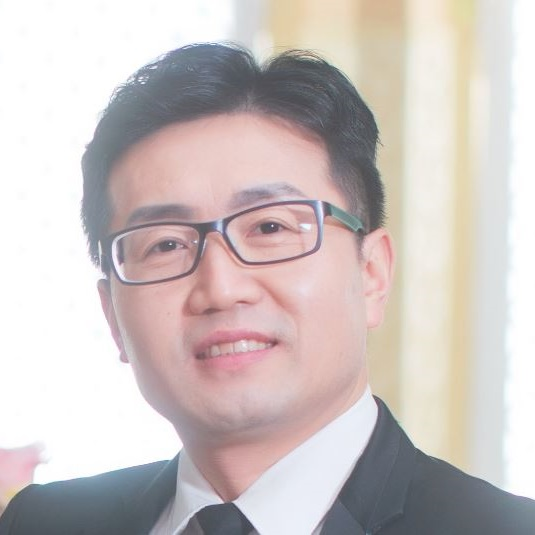C语言及程序设计进阶例程-14 开发一个电子词典

1103 0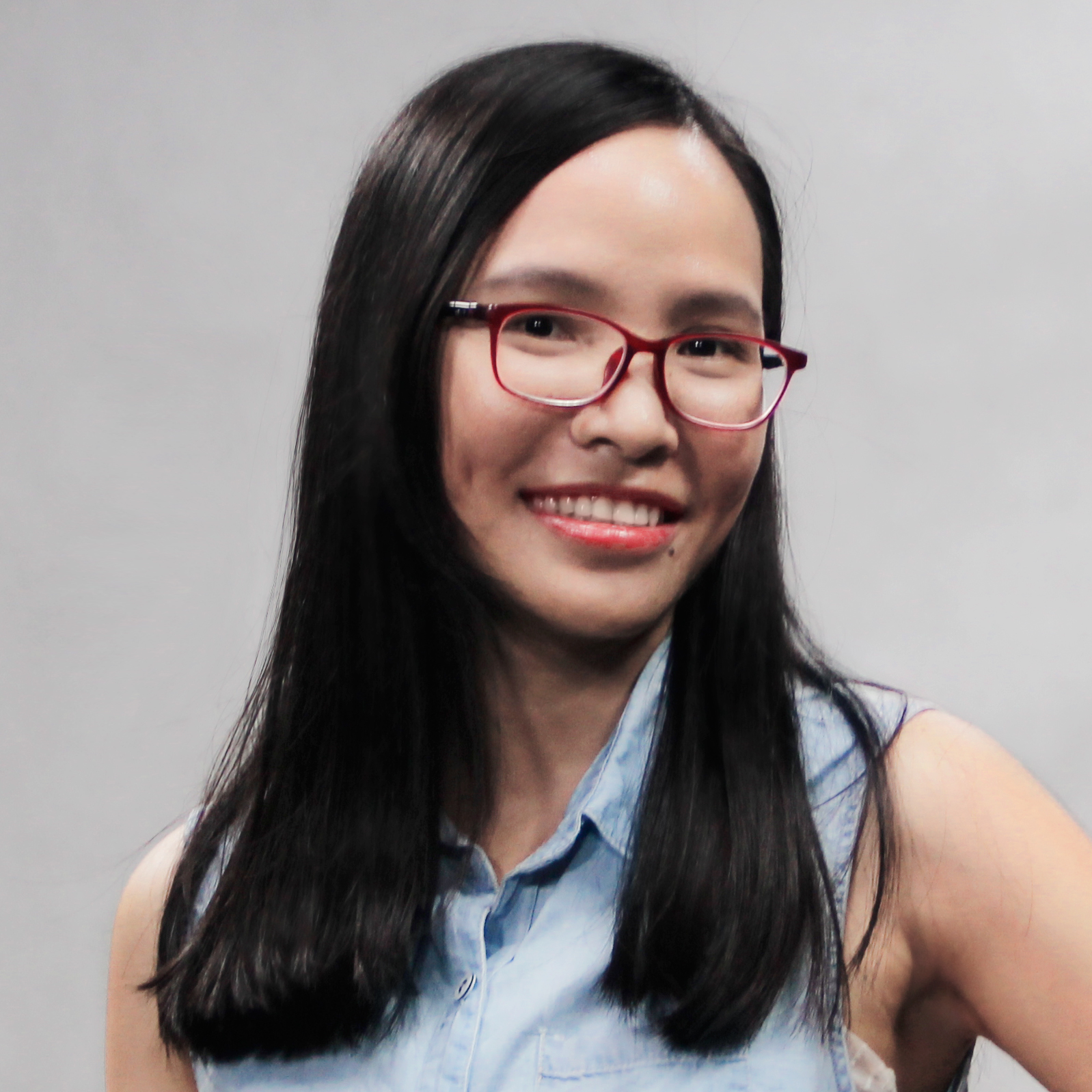MaxCompute Studio使用心得系列6——一个工具完成整个Python UDF开发
2017/12/20 北京云栖大会上阿里云MaxCompute发布了最新的功能Python UDF，万众期待的功能终于支持啦，我怎么能不一试为快，今天就分享如何通过Studio进行Python udf开发。
6866 0《测试驱动的嵌入式C语言开发》——2.3节CppUTest：一个用C++实现的自动化单元测试框架

1312 0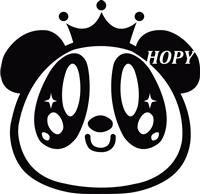RubyMotion之父：Ruby是目前替代Objective-C的最佳iOS开发语言
1494 0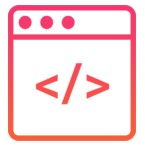C语言精要总结-指针系列（二）
此文为指针系列第二篇： C语言精要总结-指针系列（一） C语言精要总结-指针系列（二） 指针运算 前面提到过指针的解引用运算，除此之外，指针还能进行部分算数运算、关系运算 指针能进行的有意义的算术运算包括加减法运算，但不包括乘除运算。
1137 0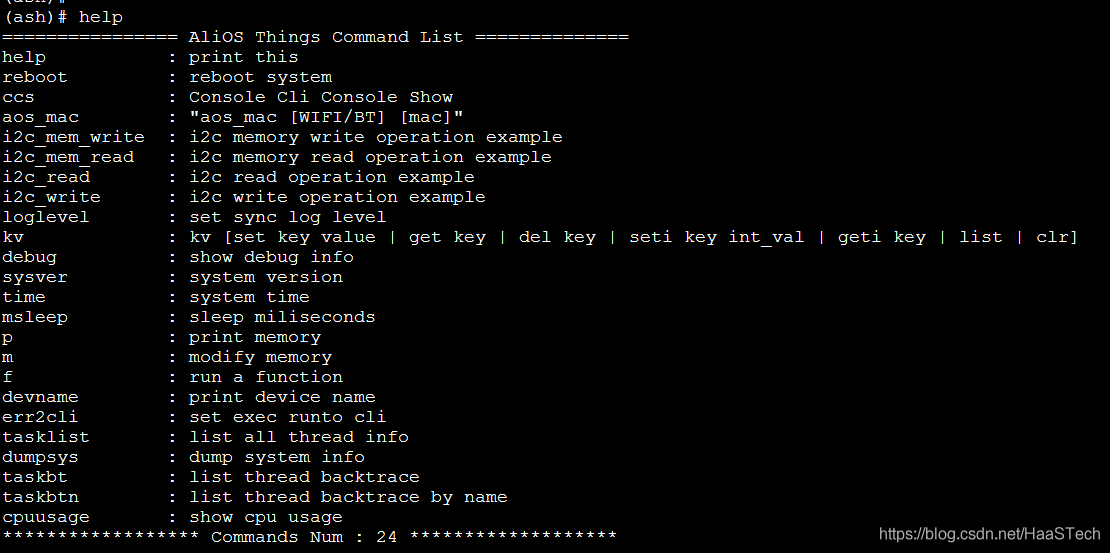HaaS100开发调试系列 之 定位AliOS Things内存及Crash问题

78 0
57

0

《2021云上架构与运维峰会演讲合集》

《零基础CSS入门教程》

《零基础HTML入门教程》